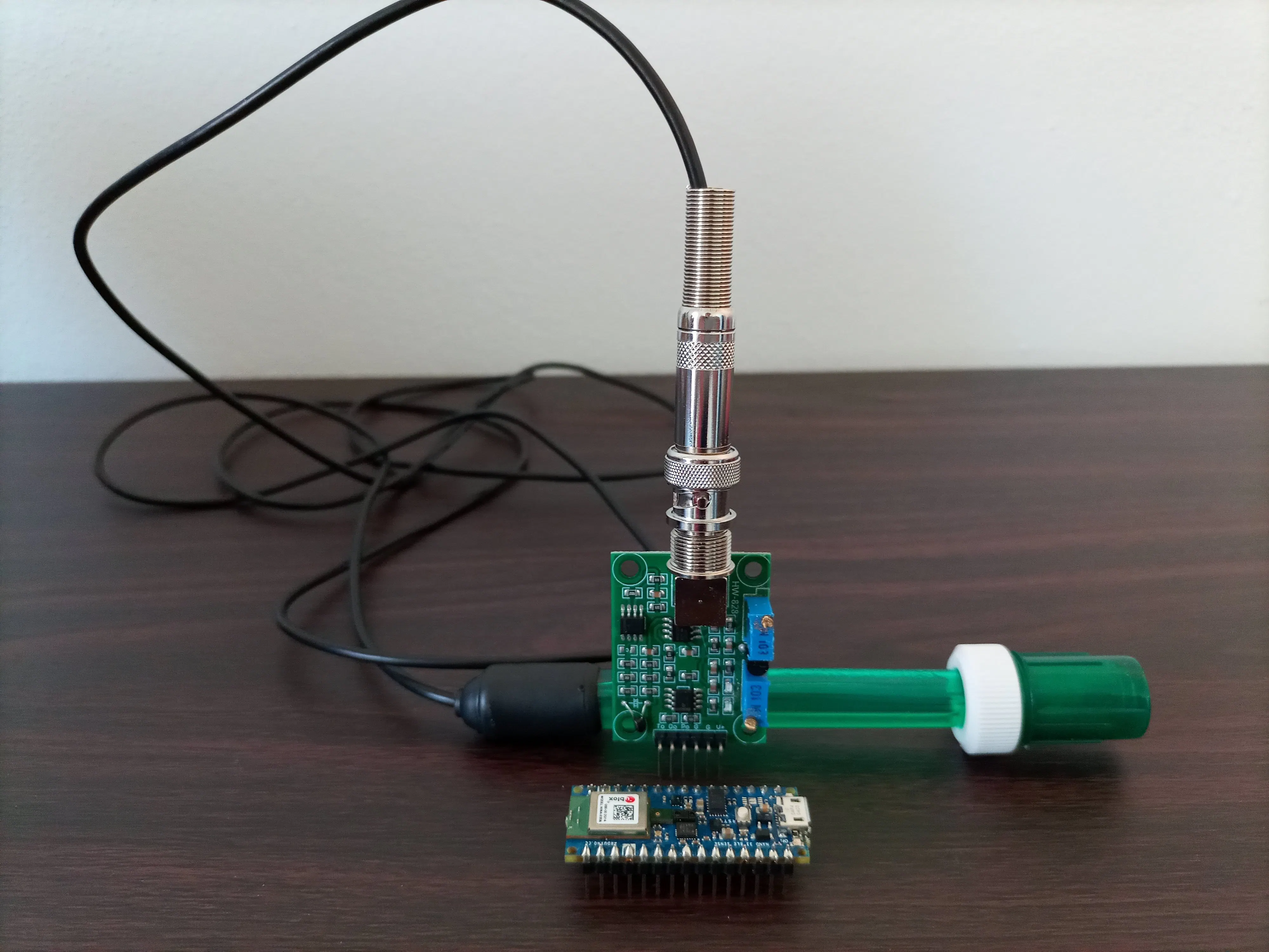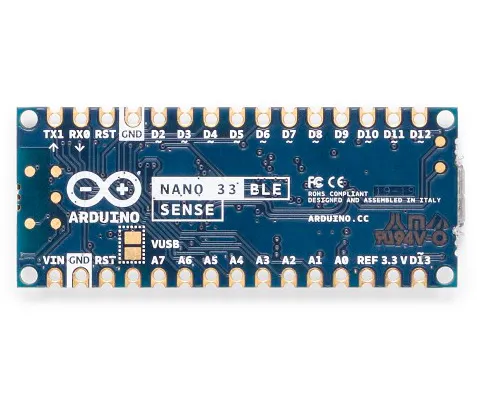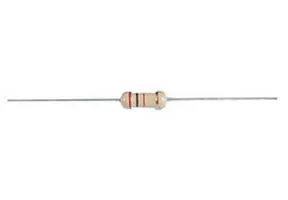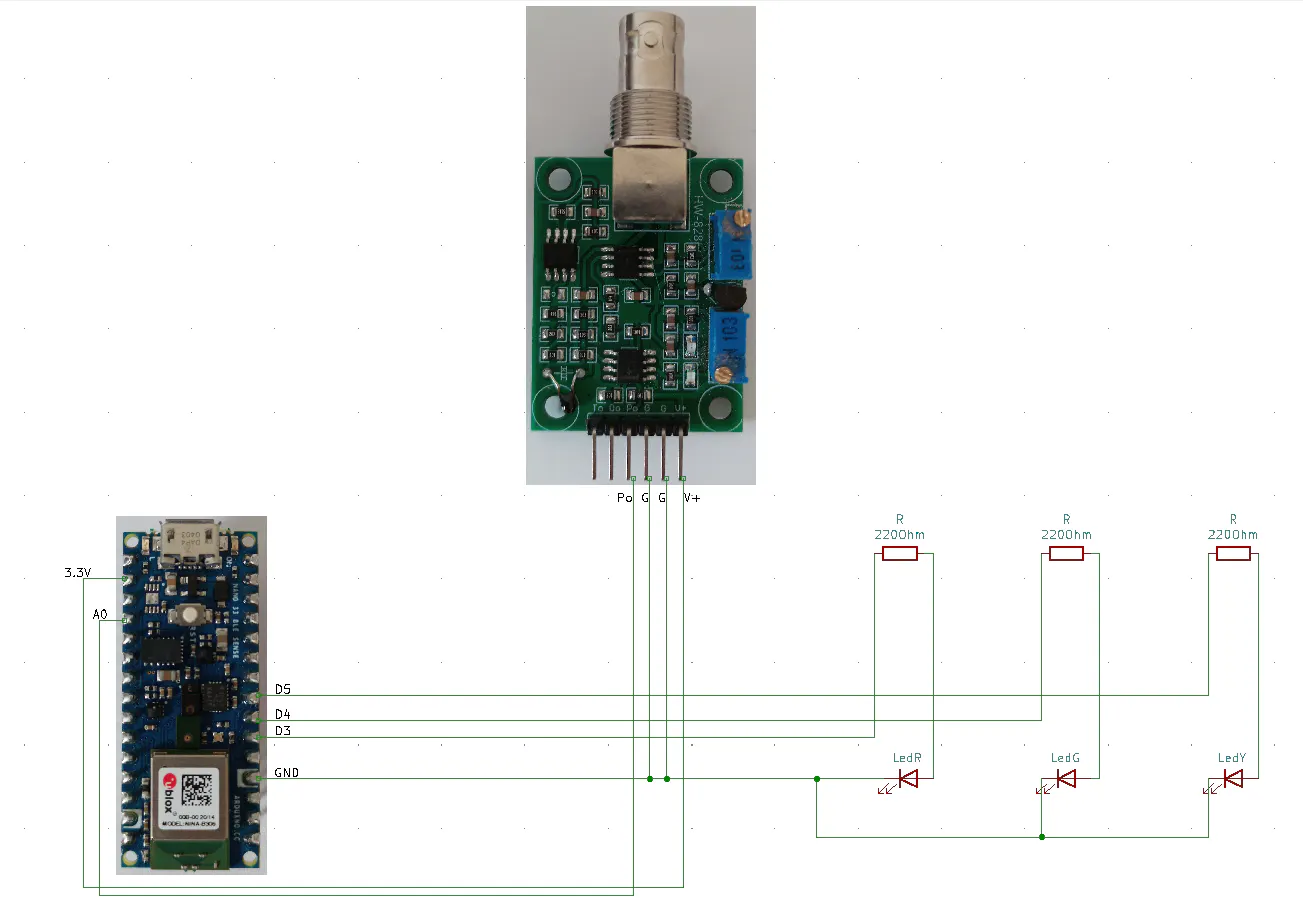# Water pH Monitoring for Hydroponic Plant

Sense the water pH of a hydroponic plant with Arduino Nano 33 BLE Sense and determine if it's right using an Edge Impulse trained model.

IntermediateFull instructions provided6 hours5,819## Things used in this project

### Hardware componentsArduino Nano 33 BLE Sense
×1
 standard ph probe
×1
 pH sensor
×1LED (generic)
×3Resistor 220 ohm
×3

### Software apps and online servicesArduino IDEEdge Impulse Studio

## Schematics

### Arduino Nano 33 BLE Sense pH sensor## Code

### pH.ino

C/C++
This code is tailored for the specific pH sensor used in the project, you might need to modify some parts according to your sensor
```/******* This code is tailored for the specific pH sensor used in the project, you might need to modify some parts according to your sensor ****************/

#include <pHmodel_inferencing.h> //library deployed with Edge Impulse
#define K 1.84f       //multiply the voltage value with this constant to obtain                       //the pH value

/****** for the data forwarder (to collect data with Edge Impulse) *******************/
#define FREQUENCY_HZ        50
#define INTERVAL_MS         (1000 / (FREQUENCY_HZ + 1))
/**************************************************************************************/

float voltage;
float ph;
int i;

/******* Leds that indicate the pH level *************/
int ledR = D3; // pH 4
int ledG = D4; // pH between 5 and 6
int ledY = D5; // pH 7
/*******************************************************/

static unsigned long last_interval_ms = 0;
// to classify 1 frame of data you need EI_CLASSIFIER_DSP_INPUT_FRAME_SIZE values
float features[EI_CLASSIFIER_DSP_INPUT_FRAME_SIZE];
// keep track of where we are in the feature array
size_t feature_ix = 0;

void setup() {
voltage = 0.0;
ph = 7.0;
/******* initialize the output pins and turn off the leds **********/
pinMode(ledR, OUTPUT);
pinMode(ledG, OUTPUT);
pinMode(ledY, OUTPUT);
digitalWrite(ledR, LOW);
digitalWrite(ledG, LOW);
digitalWrite(ledY, LOW);
/****************************************************/
Serial.begin(115200);
Serial.println("Ph meter");
}

void loop() {

if (millis() > last_interval_ms + INTERVAL_MS) {
last_interval_ms = millis();
for (i = 0; i < 10; i++) //collect some analog values from the pH sensor
{
delay(10);
}
voltage = voltage / 10.0; //median of the values
voltage = (float)voltage * (5.0 / 4095); //analog value converted in voltage value
ph = K * voltage;  //ph value

if (ph <= 1.0f) //the probe is disconnected from the sensor -> all leds on
{
digitalWrite(ledR, HIGH);
digitalWrite(ledG, HIGH);
digitalWrite(ledY, HIGH);
}

if (ph >= 8.90f) //when the voltage increase, the sensed pH increased. But the real pH measured with the indicator paper decrease -> ph = ph - 5 to obtain the real value
//if the pH is on its maximum level
{
ph = ph - 5.0;
}
if ((ph >= 8.0f) && (ph < 8.90f)) //when the voltage increase, the sensed pH increased. But the real pH measured with the indicator paper decrease -> ph = ph - 3.0 to obtain the real value
//when the pH is lower then its maximum level
{
ph = ph - 3.0;
}
Serial.println(ph, 2);
Serial.print("\t"); //it allows the data forwarder to identify the variable ph as the variable with the sensed data

/************************* run the trained model to detected the pH level *******************************/
if (feature_ix == 0) //array index
{
features[feature_ix] = ph;
feature_ix++;
}
if ((feature_ix != 0) && (feature_ix < (EI_CLASSIFIER_DSP_INPUT_FRAME_SIZE - 1)) ) features[feature_ix++] = ph;

ei_printf("Data collection. feature_ix %d EI_CLASSIFIER_DSP_INPUT_FRAME_SIZE %d\n", feature_ix, EI_CLASSIFIER_DSP_INPUT_FRAME_SIZE);

if (feature_ix == (EI_CLASSIFIER_DSP_INPUT_FRAME_SIZE - 1)) //if we are at the end of the array -> end data collection
{
ei_printf("End data collection\n");
ei_impulse_result_t result;

// create signal from features frame
signal_t signal;
numpy::signal_from_buffer(features, EI_CLASSIFIER_DSP_INPUT_FRAME_SIZE, &signal);

// run classifier
EI_IMPULSE_ERROR res = run_classifier(&signal, &result, false);
ei_printf("run_classifier returned: %d\n", res);
if (res != 0)
{
ei_printf("Cannot classify\n");
}

else
{
// print predictions
ei_printf("Predictions (DSP: %d ms., Classification: %d ms., Anomaly: %d ms.): \n",
result.timing.dsp, result.timing.classification, result.timing.anomaly);

// print the predictions
for (size_t ix = 0; ix < EI_CLASSIFIER_LABEL_COUNT; ix++) {
ei_printf("%s:\t%.5f\n", result.classification[ix].label, result.classification[ix].value);
}

if ( (result.classification.value > result.classification.value) && (result.classification.value > result.classification.value) ) //ph 4 detected
{
digitalWrite(ledR, HIGH);
digitalWrite(ledG, LOW);
digitalWrite(ledY, LOW);
}

if ( (result.classification.value > result.classification.value) && (result.classification.value > result.classification.value) ) //ph 5 detected
{
digitalWrite(ledR, LOW);
digitalWrite(ledG, HIGH);
digitalWrite(ledY, LOW);
}

if ( (result.classification.value > result.classification.value) && (result.classification.value > result.classification.value) ) //ph 7 detected
{
digitalWrite(ledR, LOW);
digitalWrite(ledG, LOW);
digitalWrite(ledY, HIGH);
}

#if EI_CLASSIFIER_HAS_ANOMALY == 1
ei_printf("anomaly:\t%.3f\n", result.anomaly);
#endif
}

// reset features frame
feature_ix = 0;
}

}

}

void ei_printf(const char *format, ...)
{
static char print_buf = { 0 };

va_list args;
va_start(args, format);
int r = vsnprintf(print_buf, sizeof(print_buf), format, args);
va_end(args);

if (r > 0) {
Serial.write(print_buf);
}
}
```

## Credits

### Janet N

1 project • 8 followers
I'm a freelance electronic engineer specialized in firmware development for embedded systems.